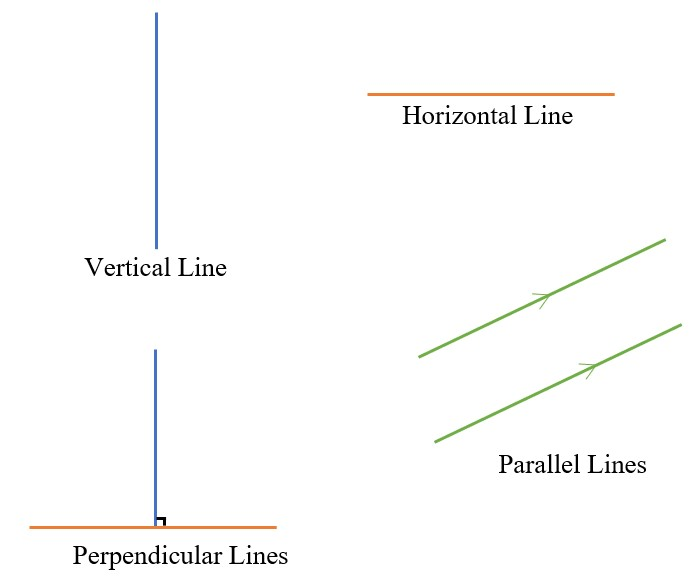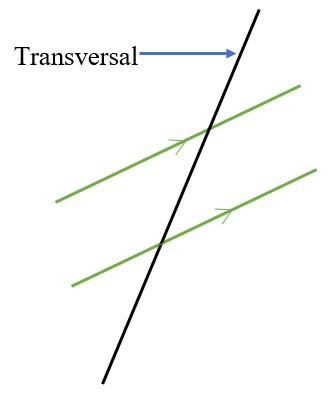4 Ijesha Close, Ilupeju, Lagos
+2347 086 296 002

#### Geometry

##### LINES AND ANGLES• Angles are formed at the point of intersection of two lines.
• Angles can be defined according to their sizes:
• Acute angle: This angle is less than 90°
• Right angle: This angle is equal to 90°
• Obtuse angle: This angle is between 90° and 180°, i.e. This angle is greater than 90° but less than 180°
• Reflex angle: This angle is between 180° and 360°, i.e. This angle is greater than 180° and less than 360°
• Complementary angles: These are angles that sum up to 90°.
• Supplementary angles: These are angles that sum up to 180°
• Perpendicular lines are lines that intersect at right angles; 90°.
•• The orientation of the lines do not matter, as long as the angle between them is 90°, they are perpendicular.
• A line that cuts across two or more parallel lines is called a transversal.• Using the diagram below to define more angles:• Angles at a point add up to 360°. Therefore, a + b + c + d = 360° and e + f + g + h = 360°
• Angles on a straight line add up to 180°. Therefore, a + b = c + d = a + d = b + c = 180° and
e + f = g + h = e + h = f + g = 180°
• Vertically opposite angles are equal. Therefore, a = c, b = d, e = g, f = h.
• Alternate angles are equal. Therefore, c = e, and d = f
• Corresponding angles are equal. Therefore, a = e, b = f, c = g, and d = h.
• Two interior angles on the same side of the transversal add up to 180°.
Therefore, d + e = c + f = 180°
• NOTE: There might be more than one way to get a particular angle. (Check out Example 1a)
##### POLYGONS
• A polygon is a plane figure that is bounded by many straight lines.
• A regular polygon has all sides and angles equal.
• A quadrilateral is a four-sided polygon. Examples, square, rhombus, kite, rectangle, etc.
• Other examples of polygons:
• A pentagon has five sides• A decagon has ten sides• The interior angles of an n-sided polygon would add up to (2n – 4) × 90° or (n – 2) × 180°• The exterior angles of a polygon would add up to 360°###### Examples
1. Determine the angles a, b, c in the following:
1.• To get c:
• 62° + c + 55° = 180° [Sum of angles on a straight line]
• ∴ c = 63°
• To get b:
• First step:
• b = 55° [Vertically opposite angles]
• Second step:
• b + 62° + c = 180° [Sum of angles on a straight line]
• b + 62° + 63° = 180°
• ∴ b = 55°
• To get a:
• First step:
• a + b = 180° [Sum of angles on a straight line]
• a + 55° = 180°
• ∴ a = 125°
• Second step:
• a = 62° + c [Vertically opposite angles]
• a = 62° + 63°
• ∴ a = 125°
2.• To get a:
• 65° + a + 47° = 180° [Sum of angles on a straight line]
• ∴ a = 68°
• To get b:
• a + b = 180° [Interior angles on the same side]
• 68° + b = 180°
• ∴ b = 112°
• To get c:
• a = Angle DEC [Corresponding angles}
• c = Angle DEC [Alternate angles]
• ∴ c = a = 68°
2. Find the values of all unknown angles below:• To get a:
• a = 40° [Vertically opposite angles]
• To get d:
• d = 55° [Vertically opposite angles]
• To get c:
• c + 55° = 180° [Angles on a straight line]
• ∴ c = 125°
• To get b:
• b + 40° = 180°
• ∴ b = 140°
• To get e:
• c + e = 180° [Interior angles on the same side]
• 125° + e = 180°
• ∴ e = 55°
• To get f:
• Sum of interior angles in a 3-sided polygon = (3 – 2) × 180° = 180°
• ∴ d + a + f = 180°
• 55° + 40° + f = 180°
• ∴ f = 85°
• To get h:
• h = f [vertically opposite angles]
• ∴ h = 85°
• To get g:
• g + e + f = 180° [Angles on a straight line]
• g + 55° + 85° = 180°
• ∴ G = 40°
• To get k:
• h + k = 180° [Angles on a straight line]
• 85° + k = 180°
• ∴ k = 95°
• To get m:
• g = m [Alternate angles]
• ∴ m = 40°
• To get p:
• m = p [Vertically opposite angles]
• ∴ p = 40°
• To get n:
• h + m + n = 180° [Sum on interior angles in a 3-sided polygon]
• 85° + 40° + n = 180°
• ∴ n = 55°
• To get q:
• n = q
• ∴ q = 55°
3. Determine the value of x in the figures below:
1.• Sum of interior angles in a 5-sided polygon = (5 – 2) × 180° = 540°
• ∴ 120° + 70° + 45° + 45° + x = 540°
• ∴ x = 260°
2.• Sum of interior angles of a 6-sided polygon = (6 – 2) × 180° = 720°
• ∴ 130° + 120° + 100° + y + 130° + 140° = 720°
• ∴ y = 100°
• y + x = 180° [Angles on a straight line]
• 100° + x = 180°
• ∴ x = 80°
4. The interior angles of a polygon add up to 1980°. How many sides has the polygon?
• Sum of interior angles of a n-sided polygon = (n – 2) × 180°
• ∴ (n – 2) × 180° = 1980°
• n – 2 = 11
• ∴ n = 13
5. Find y in the diagram below.• Sum of exterior angles of a polygon = 360°
• ∴ 5y + 2y + 4y + 3y + 7y + 3y = 360°
• ∴ 24y = 360°
• ∴ y = 15°
Minimum 4 characters